• Call Now

1800-102-2727•

# Bar magnets, definition, properties, uses, magnetic field lines, coulomb's law, practice problems, FAQs

Everyone has played with bar magnets once in their lifetime and experienced a situation where sometime it repels the other bar magnets while sometime it attracts and no matter how many times it breaks it always either gets attracted or repulsed by the other piece. Here we will discuss such important characteristics of bar magnets in detail.

• Magnets and their classification
• Bar magnets
• Properties of bar magnet
• Types of bar magnets
• Use of bar magnets
• Magnetic field lines of bar magnets
• Pole strength of bar magnet
• Bar magnet as equivalent solenoid
• Coulomb’s law of magnetism
• Magnetic field due to monopole
• Practice problems
• FAQs

## Magnets

A magnet creates a magnetic field around itself and hence exerts magnetic forces on the objects that are present inside this field. It has the ability to exert forces(attractive or repulsive) on other magnets or objects such as metals.

### Classification of magnets

Magnets are classified as artificial or natural.

• Natural magnet: These are naturally occurring magnets having low magnetic strength as compared to the artificial ones. A stone named Magnetite (Lodestone) is an example of natural magnets that was accidentally discovered by shepherds when they observed that it had attractive properties towards iron.
• Artificial magnet: These magnets are made artificially through different processes. Artificial magnets are made as per the needs and thus their magnetic strength varies according to their applications.

## Bar magnets

A bar magnet is a rectangular object piece that is completely made of iron or steel or any other substance that has ferromagnetic properties. Ferromagnetic composites or substances show magnetic properties that are permanent in nature.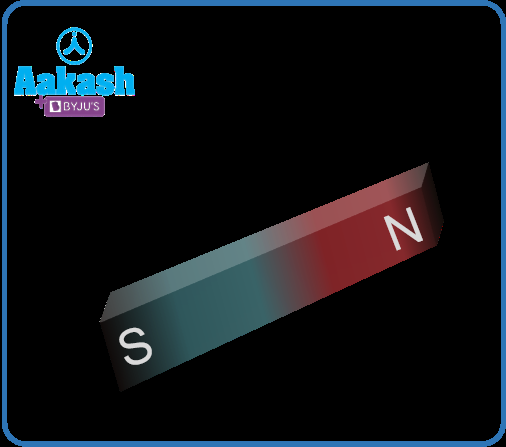## Properties of bar magnet

The following are some of the important properties that a bar magnet can possess -

• Every bar magnet consists of two poles, north, and south, at each of their ends. Even if the magnet gets broken from the middle, it will still possess the same properties. They will still have north and south poles no matter how many times it gets broken or cut.
• The magnetic force of this magnet is stronger on its poles than its middle point.
• When the magnet is suspended freely in the air tied to the thread, it would come to its original position only if the poles are arranged in a north-south position. A perfect example of this would be a mariner’s compass.
• If two bar magnets are kept together in a close manner, their like poles repel each other while their unlike poles attract one another.
• They can attract all types of ferromagnetic materials namely cobalt, steel, iron, and cobalt.

## Types of bar magnet

• Cylindrical bar magnet: As the name suggests these magnets are cylindrical in shape with length comparatively larger than its diameter. This type primarily finds its application in research and educational purposes.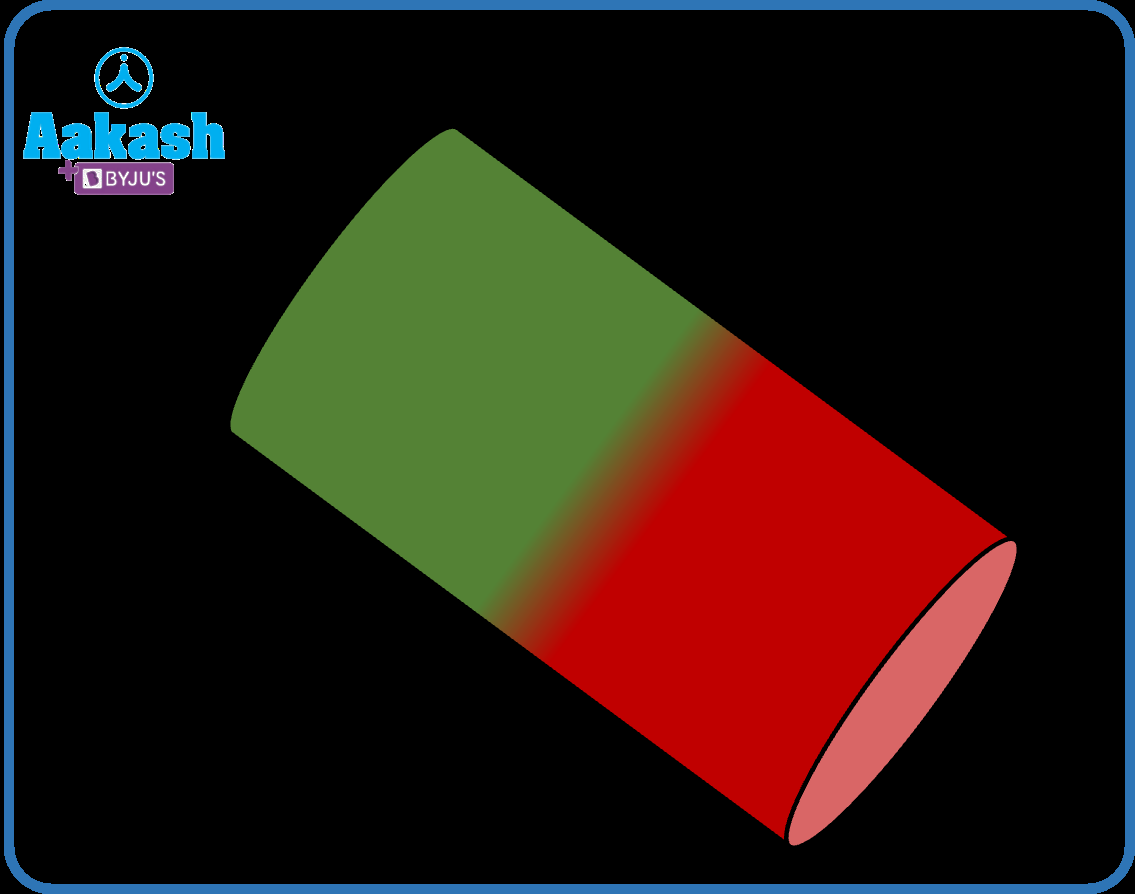• Rectangular bar magnet: These magnets resemble the shape of a rectangular bar. Due to their large magnetic strength compared to others, rectangular bar magnets find application in manufacturing industries.## Use of bar magnet

• Bar magnets can be very useful in medicine-related applications.
• Telephones, television sets, radios, and other electronic devices use magnets.
• In laboratories, long bar magnets can be utilised for magnetic experiments as stirrers.
• Various industries and factories use bar magnets to collect loose metals as well as to retain the magnetism properties of other magnets.

## Magnetic field lines of Bar magnet

Magnetic field lines present in a bar magnet are described as imaginary lines which can be simply drawn along the magnetic field. They possess certain properties,

• Continuous closed loops can be formed by magnetic field lines contained in the magnet. Outside the magnetic direction of field lines is from North pole to South pole.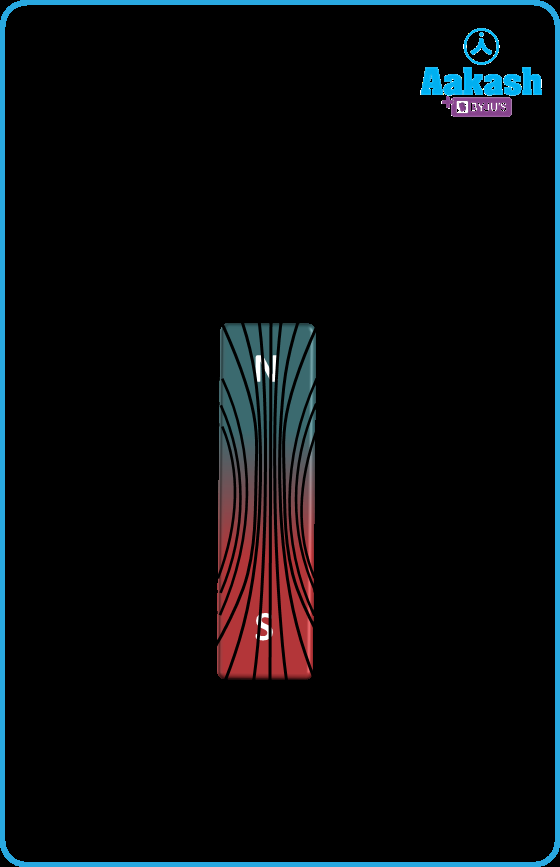• These magnetic field lines will never intersect one another.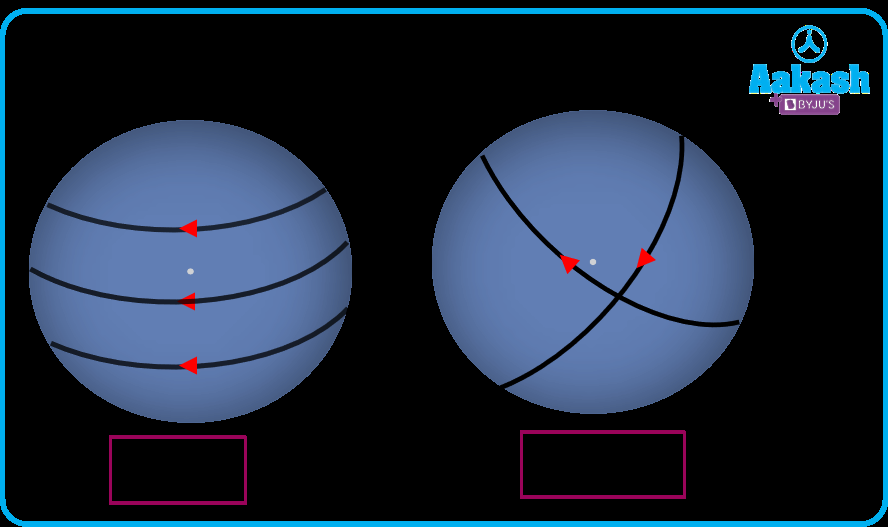• The tangent present towards the field line at any point on the magnet demonstrates the direction of net magnetic field B at that particular point.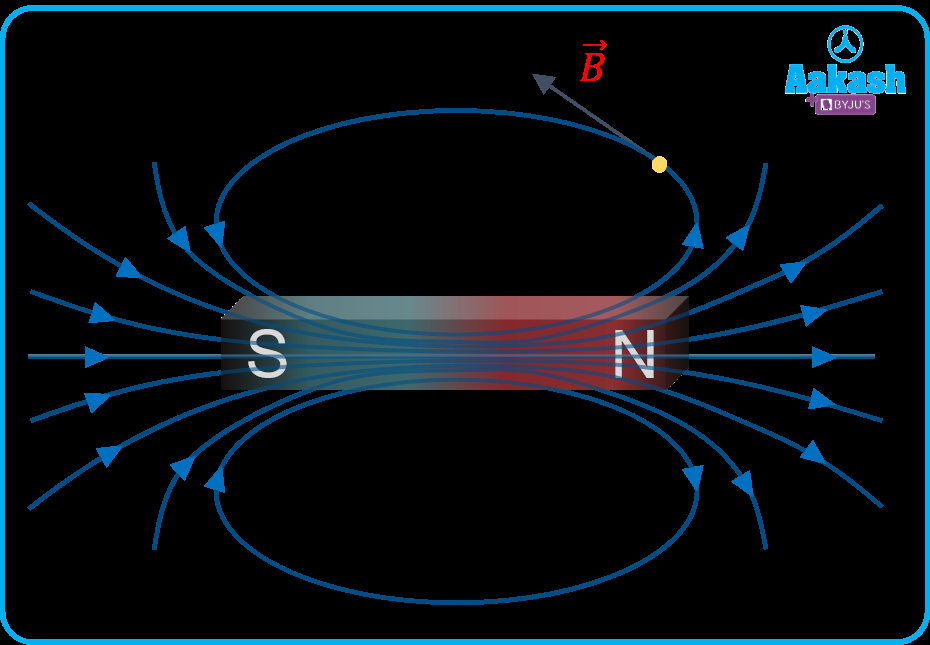• The magnitude of the magnetic field will be stronger and stronger if the number of field lines that cross the unit is larger.

## Pole strength of Bar magnet

• Pole strength represents the capability of the poles of magnet to attract magnetic material towards it.
• Pole strength is a scalar quantity.
• Just like electric charges, a magnetic charge (also called pole strength) + m is assigned to the north pole and - m to the south pole of the bar magnet.
• Unit of pole strength is either N/Tor Am.
• It depends upon the nature of the material of the magnet.

## Bar magnet as equivalent solenoid

• A solenoid is a type of electromagnet, the purpose of which is to generate a controlled magnetic field through a coil wound into a tightly packed helix.
• The resemblance of magnetic field lines of bar magnet and a solenoid suggest that a bar magnet may be thought of as a large number of circulating currents in analogy with the solenoid. It can be concluded that magnetic fields produced by a bar magnet and current carrying solenoid are quite similar.
• Also the magnetic field at large distances on the axis of the solenoid is same as the magnetic field on the axis of the ba magnet.
• Also if you cut a bar magnet in half, it will behave similarly to how a solenoid would have i.e. two solenoids having weaker magnetic properties.

### Magnetic field on the axis of solenoid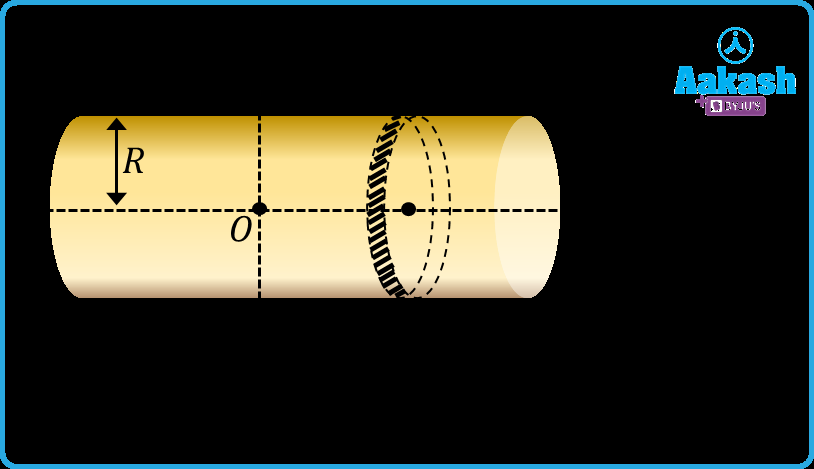Consider a solenoid of length 2L, radius R having number of turns per unit of length n with I current flowing through each turn. Let the solenoid is made up of large number of circular elements each of thickness dx.

Take a circular element (dx) at a distance x from the centre of the solenoid. This element will be having ndx number of turns.

Magnetic field at a point P on the axis is given by,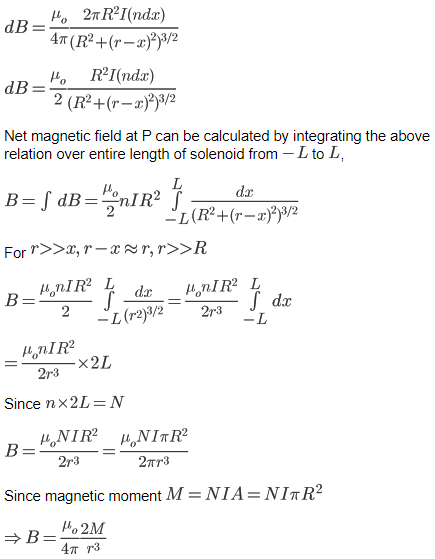This relation is similar to the magnetic field at a point on the axis of a bar magnet. Thus a bar magnet can be considered an equivalent solenoid.

## Coulomb’s law of magnetism

Let pole strength of a monopole be m, then magnetic force between two isolated poles kept at separation r is proportional to the product of the pole strength and inversely proportional to the square of distance between them.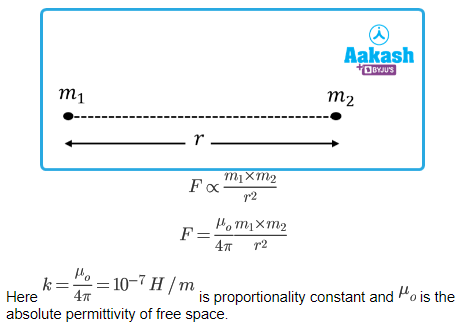• The force will be attractive if the poles are unlike i.e. one pole is North and the other one is South.
• The force will be repulsive for like poles i.e either both are North pole or both are South pole.

## Magnetic field due to monopole

Cutting a magnet into a number of pieces would give you the same number of magnets implying that monopoles do not exist. Though isolated monopoles do not exist, the idea of monopoles is quite helpful in understanding the interaction between the magnets.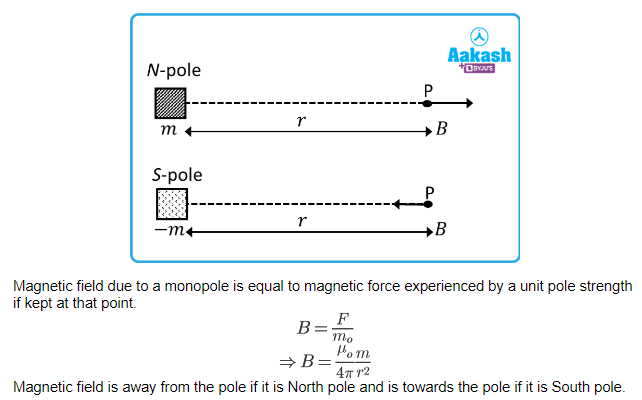## Practice problems

Q1. The repulsive force between two magnetic poles in air is 9 × 10-3 N. If the two poles are equal in strength and are separated by a distance of 10cm, calculate the pole strength of each pole.

A.

The magnitude of force between two poles are given by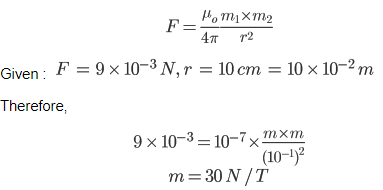Q2. The repulsive force between two like magnetic poles of equal strength are separated by the distance d in air is F. If the distance between the poles is increased to 2d, what will be the new force of repulsion between them?

A.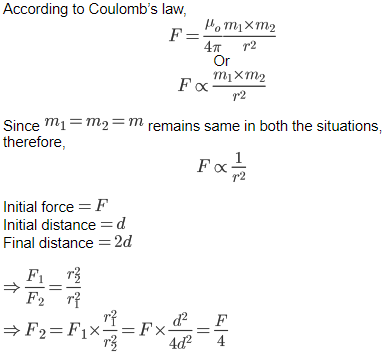Q3. Which of the following diagrams correctly represents the direction of field lines around a bar magnet?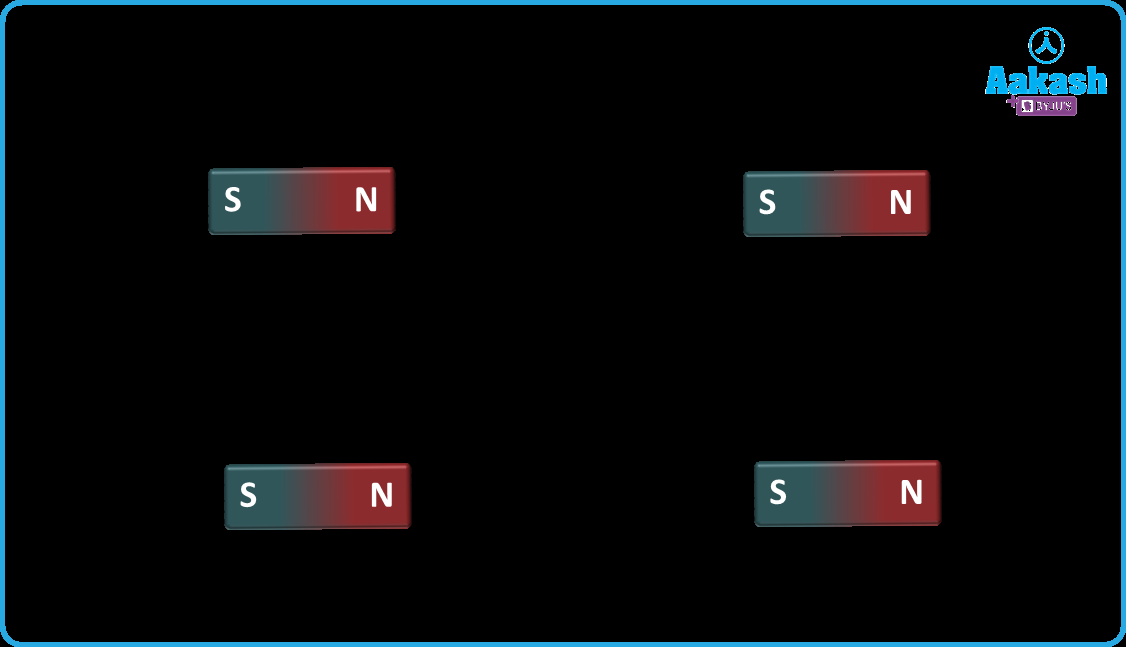A. (d)

Magnetic field line emerges from N-pole and terminates at S-pole

Q4. The diagram below represents the magnetic field near point P.If a compass is placed at same point P in the plane of magnetic field, which arrow represents the direction the north end of compass will pointA. (B)

Since magnetic field lines travel from North pole to south pole, therefore the north end of the compass will get attracted towards the direction of the south pole which is represented by option B.

## FAQs

Question 1. Why do magnetic field lines not intersect?
Answer. Because at the point of intersection two tangents can be drawn which will depict two different directions of magnetic field which is not possible.

Question 2. Can the force on unit N-pole between two isolated like poles be zero at any point?
Answer. Yes, at the midpoint of the line joining the two poles the force on unit N-pole becomes zero.

Question 3. What do you infer from the magnetic field lines around a bar magnet which are closed loops? A. Isolated poles do not exist.

Question 4. Can the force on unit N-pole between two isolated unlike poles be zero at any point?
Answer. No. At every point on the line joining them between the poles the forces due to both the poles will be in the same direction.

Question 5. Is magnetic field due to bar magnet uniform or non-uniform?
Magnetic field due to a bar magnet is non uniform since it varies both in magnitude and direction at each point inside the field.Talk to our expert
Resend OTP Timer =
By submitting up, I agree to receive all the Whatsapp communication on my registered number and Aakash terms and conditions and privacy policy